# Microgrid Optimum Identification Location using Standard and Accelerated Particle Swarm Optimization Techniques

London Journal of Engineering Research
Volume | Issue | Compilation
Authored by Samir Abood , Warsame H. Ali, John Attia, John Fuller, Penrose Cofie
Classification: NA
Keywords: APSO, PSO, and APSO, APS optimization, accelerated PSO, microgrid APSO.
Language: English

In recent years, a Microgrid has become important and commonly use. A microgrid is localized of electricity sources and loads that connect to centralized electrical power network when the need arises and disconnect to island mode. In this case, a microgrid can effectively integrate various sources of distribution generator, that can supply improving the level of voltage on the transmission line by reducing the real power losses in line and providing emergency power, also improving the voltage level at the consumer end. Accelerated Particle Swarm Optimization technique was applied in this paper, it is a modern technique appeared for optimization ,  and was compared with standard Particle Swarm Optimization technique to perform the optimization operation of power flow in transmission line by selection the optimum location of microgrid installed in power network considering minimum power losses with optimal operation consideration the no. of iteration, execution time of program and capacity. The results of simulation obtained for optimization the nonlinear objective functions using MATLAB program designate that APSO accomplishes roughly developments in terms of computation time and best fitness.

# Microgrid Optimum Identification Location Using Standard and Accelerated Particle Swarm Optimization Techniques

## ABSTRACT

In recent years, a Microgrid has become significant and commonly use. A Microgrid has localized of electricity sources and loads that connect to centralized electrical power network when the need arises and disconnect to island mode. In this case, a microgrid can effectively integrate various sources of distribution generator, that can supply improving the level of voltage on the transmission line by reducing the real power losses on it and providing emergency power, also improving the voltage level at the consumer end. In this paper, the Accelerated Particle Swarm Optimization technique was applied, it is a modern technique appeared for optimization ,and was compared with standard Particle Swarm Optimization technique to perform the optimization operation of power flow in transmission line by selection the optimum location of microgrid installed in power network considering minimum power losses with optimal operation consideration the no. of iteration, execution time of program and capacity. The results of simulation obtained for optimization the nonlinear objective functions using MATLAB program designate that APSO accomplishes roughly developments regarding computation time and best fitness.

Keywords: APSO, PSO, and APSO, APS optimization, accelerated PSO, microgrid APSO.

Author α σρѠ¥: Electrical and Computer Engineering Department, Prairie View A&M University.

1. ## INTRODUCTION

A microgrid is an isolated power system that can manage intelligent distribution power resources, interconnected loads, and can work in parallel with or independently of the network. In the same mood, electric power can be provided economically, while enhancing the quality and reliability of energy by integrating and improving energy sources. However, the validity of a microgrid network depends on the combination of power sources, size or capacity allocated and the transmission strategy . Wind energy is a promising technology commonly used in microgrid networks [2,3] Since the Winds are a clean energy source that is available everywhere and is available free of charge, but it depends to a great extent on on-site meteorology .

To minimize the loss, a microgrid site has a key role. The losses are reduced to a minimum amount by increasing the size of the installed generator [6,7]. The selection of the suitable site of distribution generator in the system also plays a main role in reducing the active power losses , interactive and interactive. In this paper, many investigations have future different methods to decrease losses of the system. A Distribution Generator (DG) can also reduce the cost of the network upgrade, however, if the location of  distribution generator is not realistic, on the conflicting, may lead to an increase energy loss, so, the preparation and scaling size of distribution generator depends on the minimum transmission loss; send traditional power system,and power transmission distribution is managed together .

The main features affecting the cost of energy transfer losses are transmission line losses and the generating price. In this situation from the specified energy flow, all generations prices are Basically the same in the traditional power grid, so, The minimum loss of transmission line means almost The minimum cost of transmission losses; but the cost of generating DG is much higher than traditional generations, location DG measurement may only consider loss of transmission to increase the transmission cost in the power grid layout, the calculations are optimized with the cost of transmission loss goal function is necessary .

In this research, we proposed a schematic  for the loss reduction system, the 4-step loss reduction plan, as described below, includes a divided into sections including analysis of the load flow, acquisition of the voltage profile, control, and reduction of losses and optimization using an approach based on two algorithms are obtained for the placement of DG, the PSO, and APSO, and monitor the data profile .

1. ### STANDARD PARTICLE SWARM OPTIMIZATION TECHNIQUE (PSO):

In the year 1995, J. Kennedy and R. Eberhart have developed the PSO technique, based on the attitude of a swarm such as bird and ﬁsh education in Nature. There are at least twenty variants of PSO technique, and hybrid algorithms were combining by PSO technique with other remaining algorithms. A PSO technique exploration for object planetary by regulating the paths of individual factors, called particles, as cross-sections made up of topical vectors in a semi-stochastic manner. Article movement factors consist of two main components: an unavoidable element and a random component. Each particle is attracted to the best current position G and the bestsite in history, while at the same time tends to transfer arbitrarily. Let be the vector location xi and the particles velocity vi  of  i, respectively so the new speed transducer is calculated by the following formula:(1)

Where each the random vectorsandvalues are taken between (0 to 1). The acceleration parameters constants α and β are the of or learning parameters, which can usually taken as (α ≈ β ≈ 2). The most well-known optimization is most likely to use inertial function θ(t) so thatis replaced byas given:(2)

And the update of the position is given as:(3)

Where θ(t) ∈ (0,1) [2, 3]. For stabilize particle movement, and the algorithm converges faster Usually taken θ(t) about between 0.5 to 0.9.

1. ACCELERATING PARTICLE SWARM OPTIMIZATION TECHNIQUE (APSO)

When required the diversity in quality solutions increase, improving the standard PSO uses both the best position individual. And the global best g*. A diversity was simulated by using randomization for using the best individual is mainly. Later, there is no convincingly motive to use the best individuals, except the problem optimization is multimodal and extremely nonlinear. A basic model that can accelerate the meeting of the procedure is to use the globally better only. Thus, in this way of the acceleration the of particle swarm optimization [11, 12], the velocity vector is generated by equation:(4)

Where:is outgoing N (0,1) to replace the next term. To increase the convergence more, we can also write the location update in one step:(5)

Usually, α = 0.1K to 0.5K where K is the measure of each variable, and β select from 0.1 to 0.7 for suitable in most applications. There is no requirement to accord with the vectors of velocity formation, so be noted that speed does not appear in equation (5) therefore APSO is much simpler, and the mechanism is easy to understand. Compared with many standard PSO variables, the APSO uses exclusive two parameters, another improvement in the accelerated PSO system is to reduce randomization while duplicates persist by using a monotonous decrease function like:

α = α
0.exp[-γt]                            (6)

or

α = α0 . γt ,    (0 < γ < 1)                      (7)

Where: α0 approximately from 0.5 to 1 is the initial value of the random parameter. Here t is the number of duplicates or time steps, and γ  is a control parameter . For example, in this application, we will use:

α = 0.75t                                 (8)

Where: t ∈ [0 and Tmax] and Tmax is the maximum iteration .

1. ### OBJECTIVE FUNCTION

In the power systems, the dispatch of reactive electricity power may have different goals, such as obtaining the best voltage quality, reducing the real power losses. Considered the voltage magnitude is |Vi| and |Vj| at buses i and j respectively so, the power loss as an objective function and described by the formula:(9)

Where M is the number of branches, while the angle θij is the difference in phase of voltage angle at bus i, and bus j respectively, and gij represent the branch conductance of impedance between the buses i and j.

### V.   CONSTRAINTS

There are two constraints dispatch issue of reactive power, equality and inequality constraints as follows:

i- Equality Constraint: If we suppose the real and imaginary power supplying  to bus i are Pgi and Qgj respectively, and the real and imaginary demand load power at bus i are Pdi and Qdi respectively, so  The real and imagery power is given by the following formulas:(10)(11)

ii. Inequality Constraint: It’s the voltage magnitude range value, tap changing transformer positions value, and injection reactive power to the system. In this paper and based upon the data of the IEEE 30-buses system, the voltage magnitude values were selected from 0.950 p.u to 1.10 p.u, and the tap changing transformer range is usually from 0.975 to 1.025. The compensators injection of reactive power to the system from 0 MVAR  to 20  MVAR. The inequality constraint was given by the following relations[14,16]:(12)(13)(14)

So, the objective function given in Equation 9 can be expressed by follows:(15)

And the values of voltage magnitude, tap changing transformer, and injection reactive power described as follows:(16)(17)(18)

1. ### SYSTEM UNDER STUDY

This paper examines the possibility of using wind power energy for electricity generation at optimum conditions and simulation network for an IEEE 30 -buses, which consists of 30 busbars was connected via 38 transmission lines, is considered for this study. The objectives are to identify more economic technology and the environmental merit of the wind microgrid.

The estimated electrical real power generation of 300 MW while the valued electrical load The consumption of    285 MW. A MATLAB program was used to analyze the system they allow comparison with different design options.

The proposed technique is performed and verified on the IEEE 30 busbars network. The power system network construction of 30-buses was indicated in Figure 1. In this network, there are 6-generators in the system, one on the bus slack and the other on the buses 2,5,8,11, and 13 respectively. There are 4-tap changer transformers between buses (4,12), (6,9), (6,10) ,and (27.28). There are 2-synchronous capacitors on bus 10, and bus 24 respectively.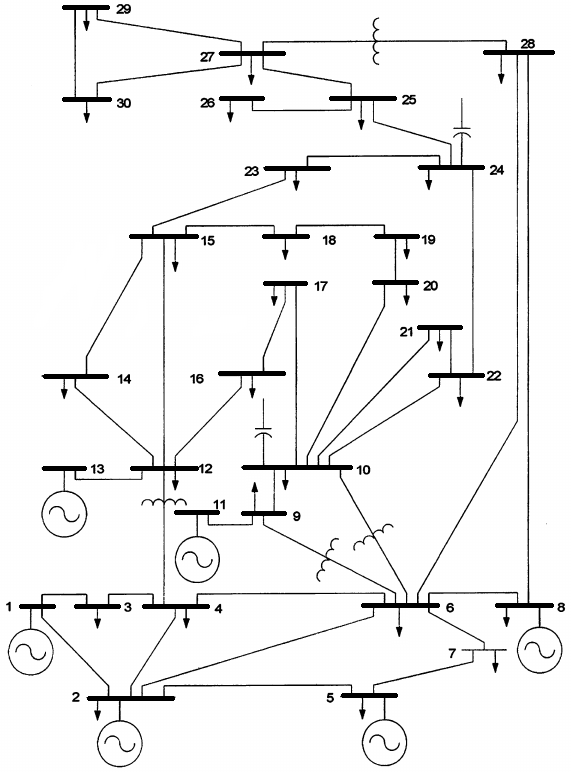Figure 1:  The IEEE 30 bus system

1. ### Technical speciﬁcations E-126 ENERCON wind energy converters

ENERCON wind energy converters run with a special storm control feature. A slows the wind turbine down so that it can continue to operate even at high wind speeds. Numerous shutdowns which lead to considerable losses in power output can thus be avoided. In this paper connected A E-126 ENERCON wind energy converters as DG with specifications given in table 1. Figure 2 illustrates Power-Wind speed characteristics for 7.5 MW /E-126 ENERCON wind energy converters, while Figure 3 shows the characteristics of Power Coefficient-Wind speed for it’s.

Table 1: Technical speciﬁcations of E-126 ENERCON wind energy converters

 Rated power 7500 kW diameter of Rotor 127 m Hub height 135 m blades No. 3 The swept area in m2 12,668 Rotational speed Variable, 5 - 12.1 rpm Cut-out wind speed: (with ENERCON storm control*) 28 - 34 m/s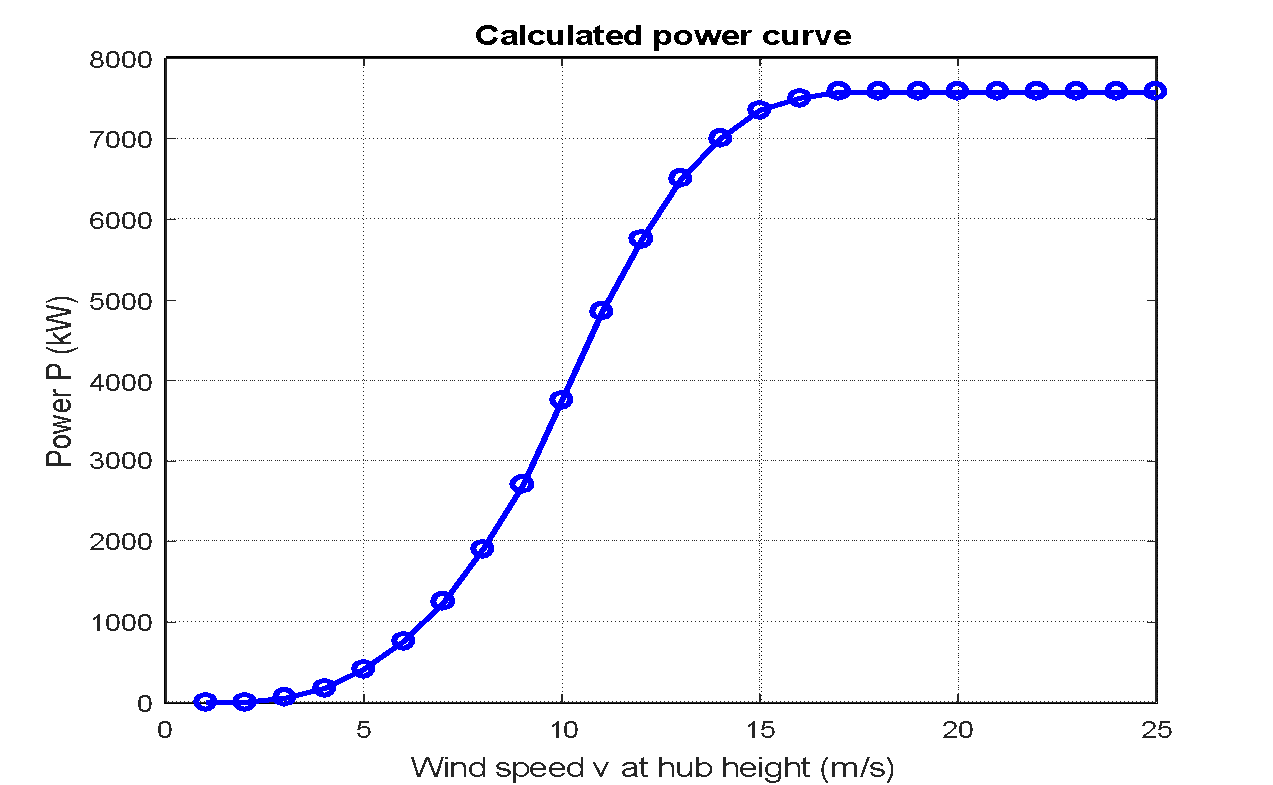Figure 2: Power-Wind speed characteristics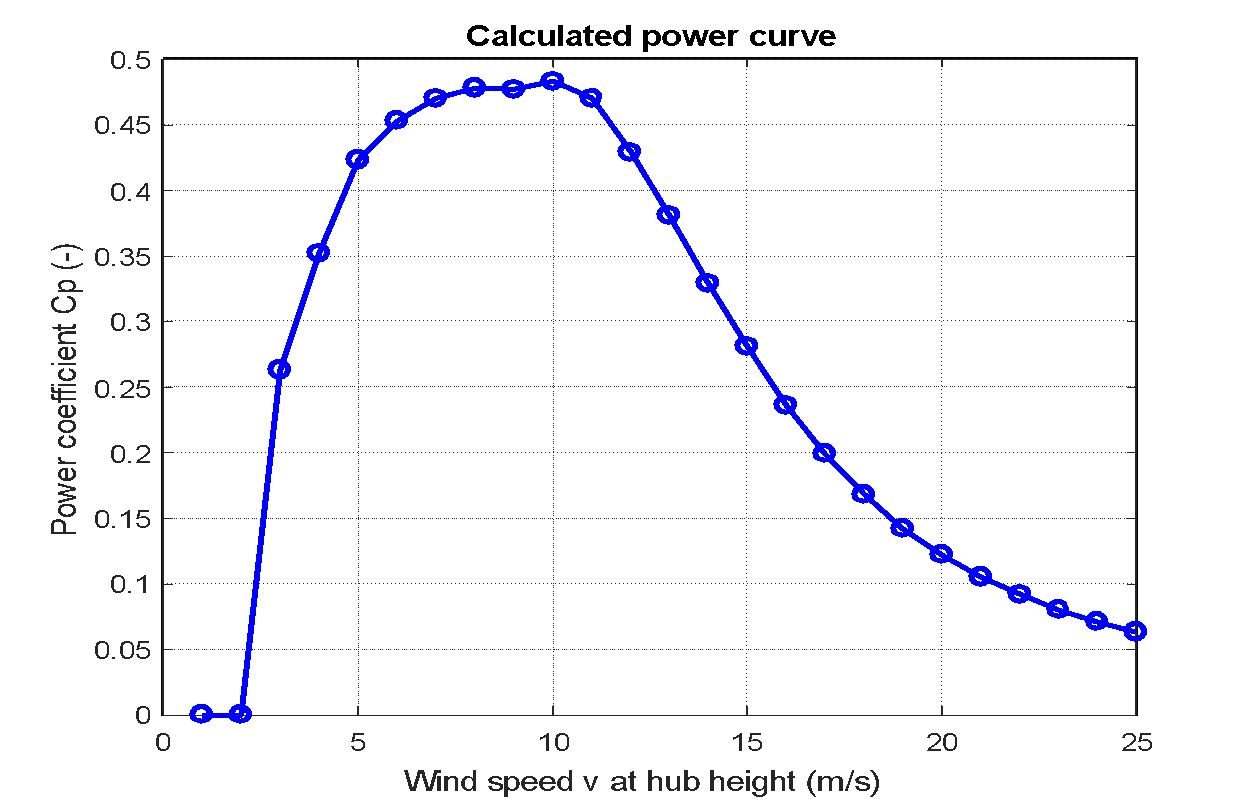Figure 3: Power Coefficient-Wind speed characteristics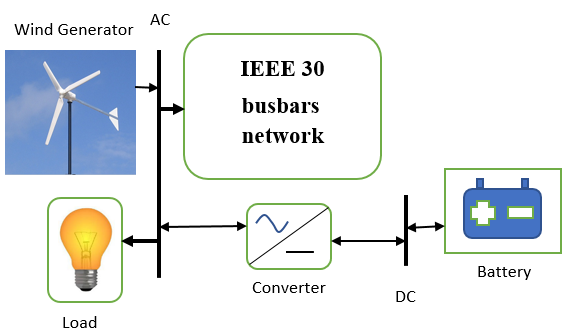Figure 4: Connecting Wind generator to the network

1. ### SELECTION PARTICLES OF PSO AND APSO

In this paper, the particle selected in each technique as the voltage magnitude at slack bus and each P|V| generation bus, also tap changing transformer ratio and the injected reactive power to the system. So there are 12 different particles are (|V1|, |V2|, |V5|, |V8|, |V11|, |V13|)  form slack and P|V | magnitude voltages, tap changing transformer ratio (T4,12, T6,9, T6,10, T27,28 ) between buses 4-12, 6-9, 6-10, and 27-28  and injected reactive power (Qc10, Qc24 ) at buses 10 and 24. When the optimization development process begins, each particle position will be a continuous update until reach the stop criteria.

The minimum inertia weight was selected as 0.45, and the maximum weight limitations were selected as 0.95. With continuous repetition, the weight value will drop from 0.95 to 0.45.

1. ### RESULTS AND DISCUSSION

In this section, we show all the results obtained from running the MATLAB program for standard PSO and APSO techniques:

Figure 5 shows the process of optimizing interactive power transmission with Installation of wind generator at the optimum location of bus no. 24, for the PSO technique, when the optimization is starting development, the position of the particles are chosen arbitrarily. The global real optimum power loss is 16.58 MW, then the particles constantly update their attitudes towards the better solution, the loss of active power remains to decline. Later 45 iterations, there can be no clear upgrading observed. Finally, the loss of real power is close to 15.15 MW.

Also, figure 6 illustrates the optimizing process interactive power transmission with Installation of wind generator at the optimum location of bus no. 15, for the APSO technique, at the starting of the optimization, the particles locations are chosen arbitrarily. The loss of active power is about 14.1 MW. When the particles update their attitudes towards the best solution, also the loss of real power remains to decline, and after 2 iterations, the loss of active power is close to 13.69 MW.

Figure 7 shows the actual power loss per branch before and after installed DG at the optimum location by using PSO and APSO techniques. This figure illustrates the loss of active power in most lines slightly decrease, (as branches from 1 to 6) when using APSO technique so the total real power loss of 30 the bus system was greatly reduced and the higher losses in each branch when using PSO without wind turbine generator.

Figures 8 and 9 show the power losses  when installing the wind generator located in a different position of the bus then it shows the minimum power, where for case PSO technique the minimum power accord when the DG located at bus 15 and the power losses of 15.15MW, And for case APSO technique the minimum power accord when the DG was located at bus 24 and the power losses of 13.157MW. So, this illustrates that the power losses reduce when using APSO technique, Figure 10 shows the difference in power losses when installing the wind generator located in a different location of the bus when using PSO and APSO techniques.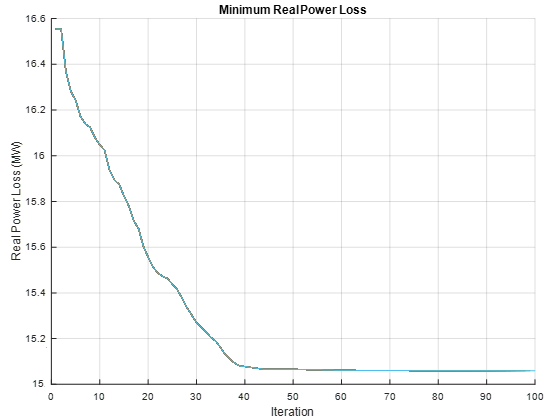Figure 5: Minimum power losses when using PSO technique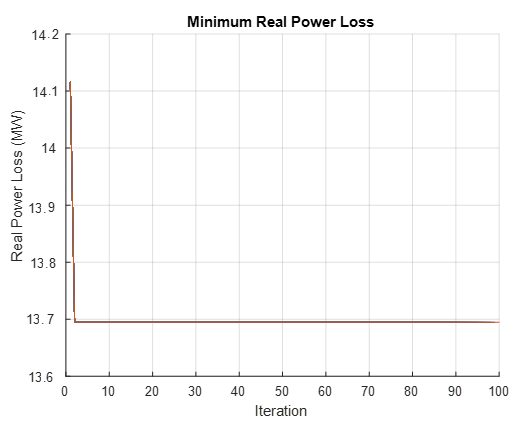Figure 6: Minimum power losses when using APSO technique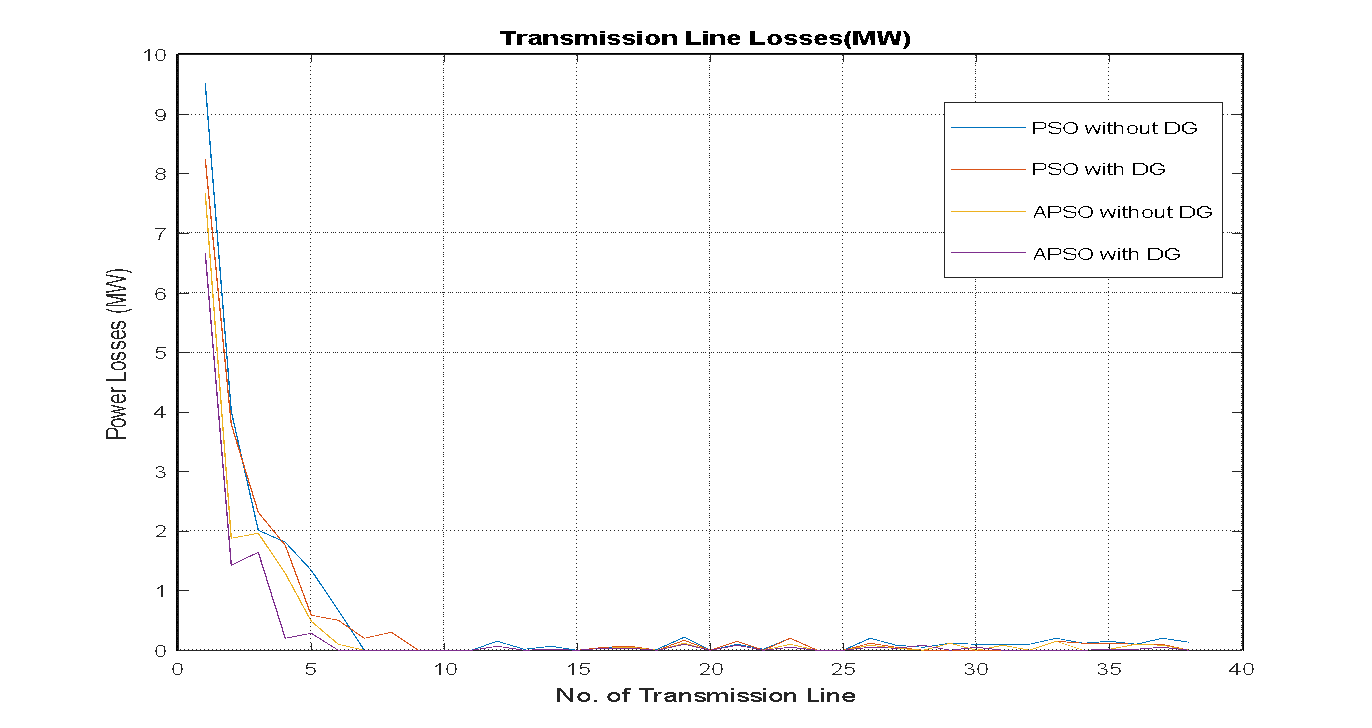Figure 7:  The Real Power Loss at Each Branch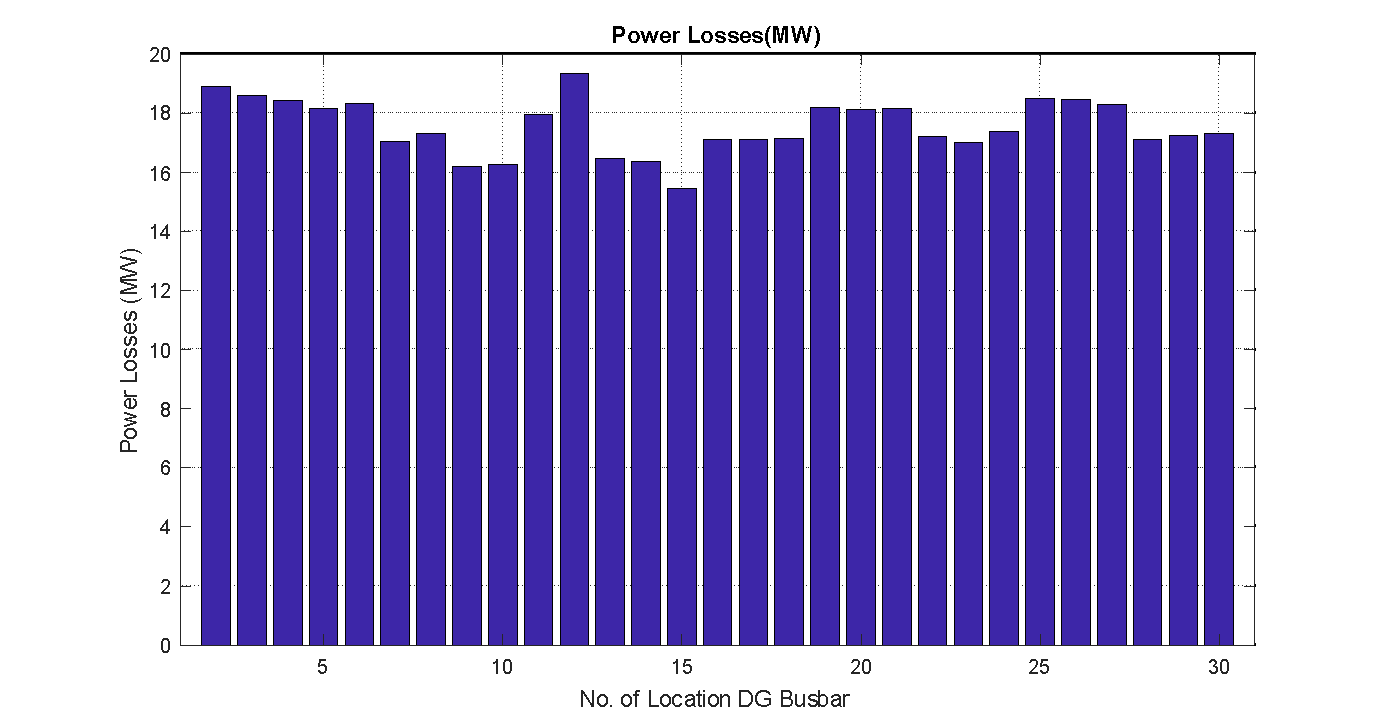Figure 8:  Active power losses when using the PSO technique when location DG at different busses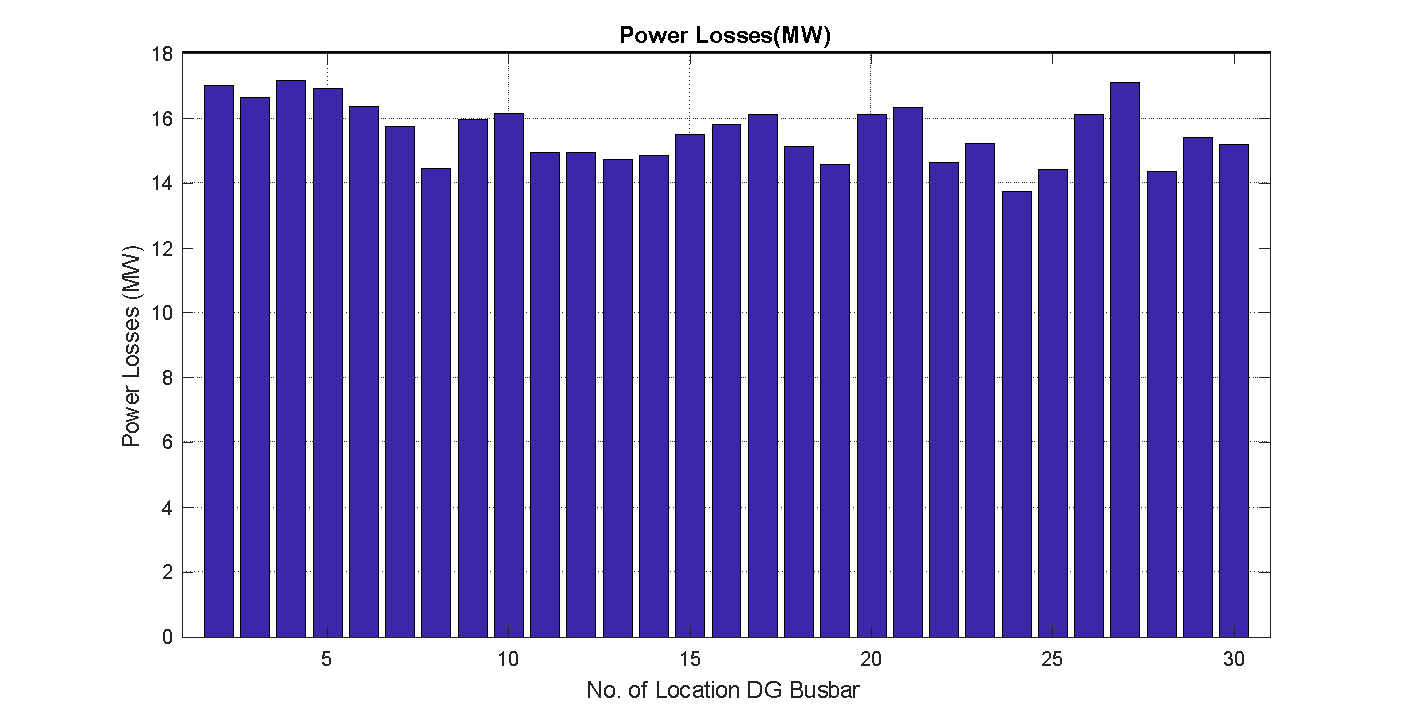Figure 9: Active power losses when using the APSO technique when location DG at different busses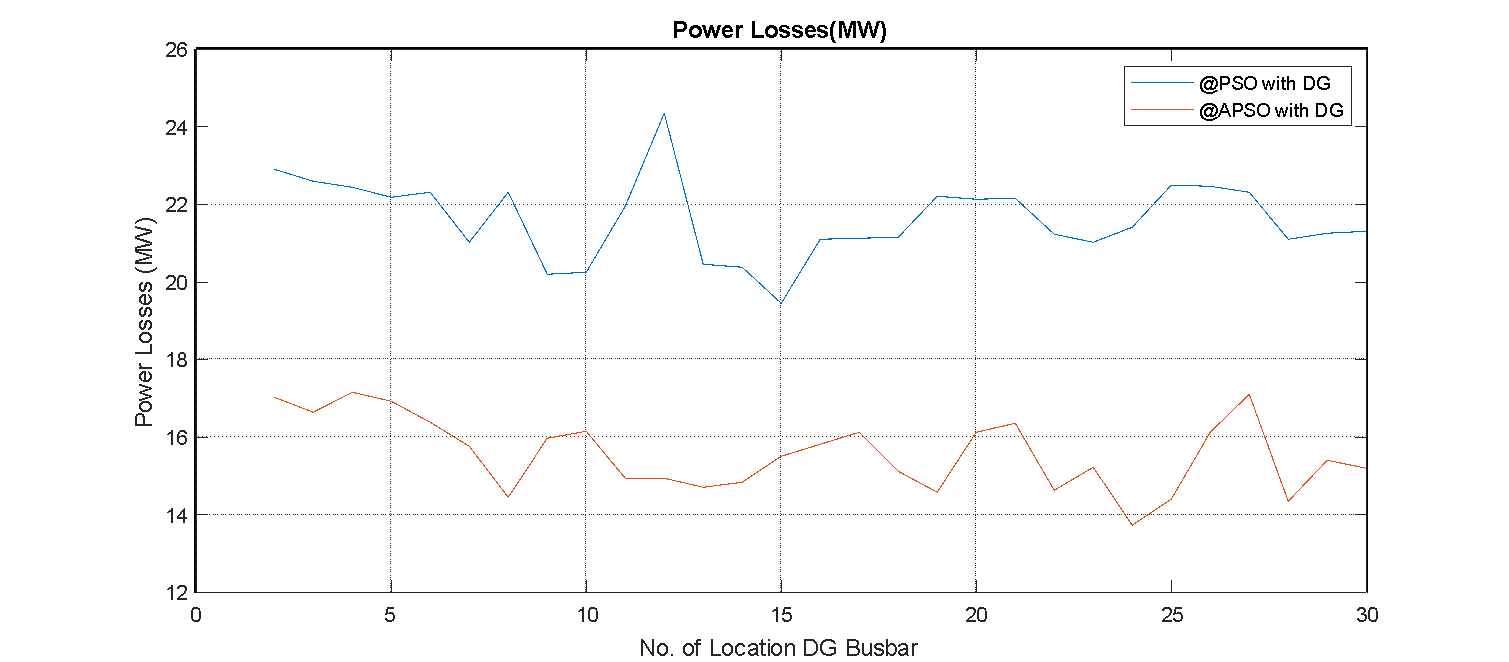Figure 10: Active power losses comparison when using PSO and APSO technique when location DG at different busses

The voltage profile was evaluated using The Fast-Decoupled Load Flow (FDLF) method then calculate the real power loss of the system. After that put wind generator at each bus to estimate the total corresponding real power losses to obtain the optimal location of the DG when the total loss accord. This paper provides a new technique proposed solution to determine the optimal location of the DG in the power system. The proposed approach is based on the APSO technique and aims at the identification of the position of the load is different and compare the results with the applied standard PSO technique. Figure 11 shows the magnitude voltage profile for different cases for PSO and APSO techniques with and without, the figure illustrates the voltage magnitude will be improved when using APSO with an installed wind generator. Also, Figure 12 explain the voltage phase angle also modified.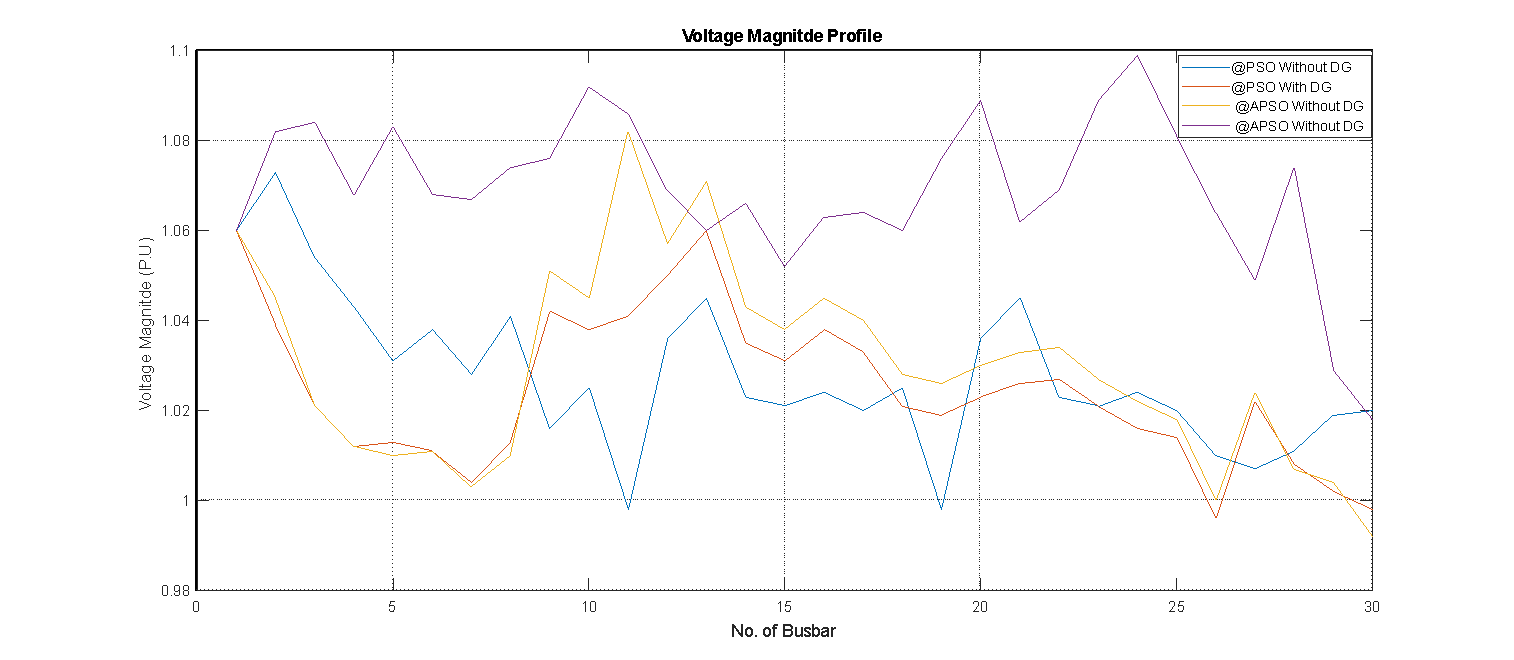Figure 11:  Voltage magnitude profile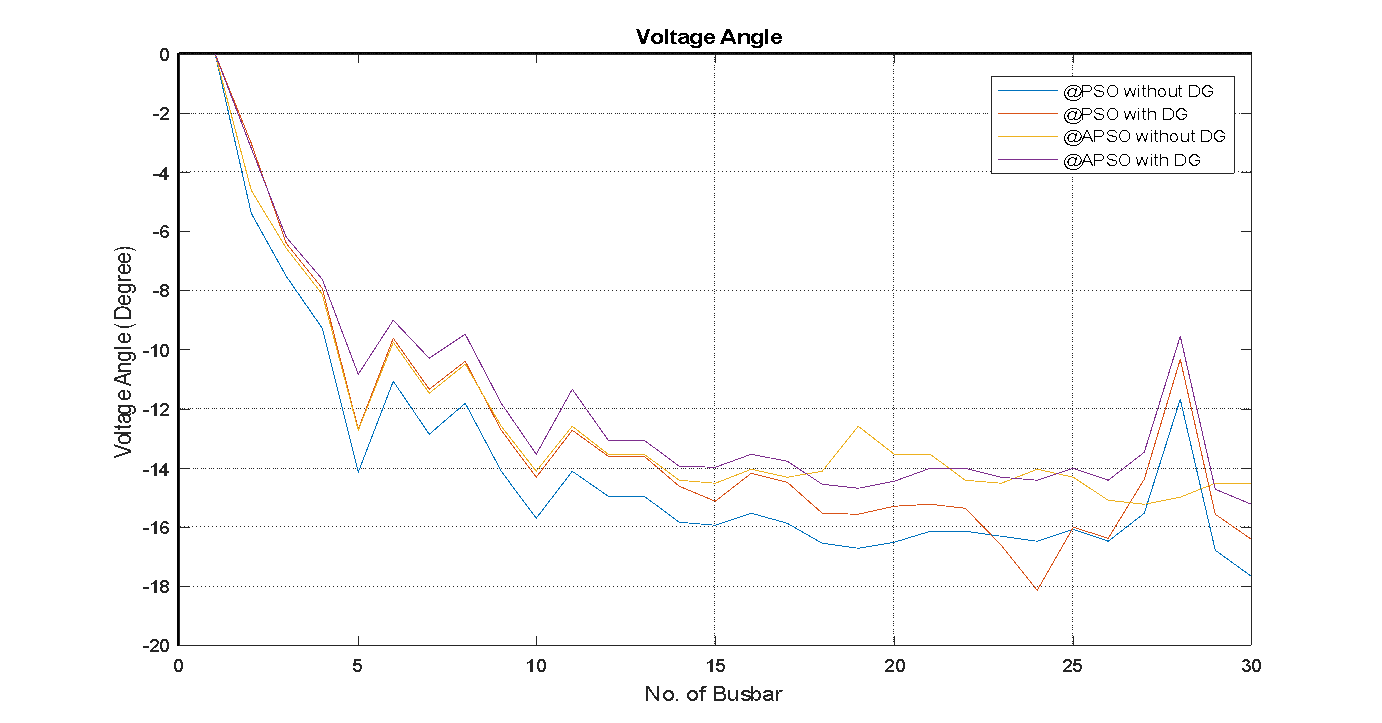Figure 12:  Voltage angle profile

### X.  CONCLUSIONS

In this paper, the comparison between two techniques are used to identification of an optimum location by using standard particle swarm optimization technique and accelerated particle swarm optimization technique; the obtained results explain the affiant the APSO method when taking the consideration of iteration number, fast of execution time, reducing power losses, improve the power losses on the transmission line and optimize the power flow, and improve the magnitude and angle voltage profile.

### REFERENCES

1. Rahman, P. Vasant, B. Singh, and M. Abdullah ”On the performance of accelerated particle swarm optimization for charging plug-in hybrid electric vehicles”, March 2016, AEJ - Alexandria Engineering Journal 55(1):419–426.
2. W. C. Zhou, Z. Lou, L. Lu, and H. Yang,                “ Current status of research on the optimum sizing of stand-alone hybrid solar-wind power generation systems,” Applied Energy, vol. 87, no. 2, pp. 380-389, 2010.
3. M. S. Okundamiya, J.O. Emagbetere, E. A. Ogujor, “ Design and control strategy for a hybrid green energy system for mobile telecommunication sites”, Journal of Power Sources, vol. 257, pp. 335-343, 2030.
4. M. S. Okundamiya, and A. N. Nzeako, “Model for optimal sizing of a wind energy conversion system for green-mobile applications,”, Int’l J.  of Green Energy, vol. 10, no. 2, pp. 205-218, 2013.
5. M. S. Okundamiya and O. Omorogiuwa,” Analysis of an Isolated Micro-Grid for Nigerian”, Terrain 2016 IEEE 59th International Midwest Symposium on Circuits and Systems (MWSCAS), Abu Dhabi, UAE, 16-19 October 2016.
6. M. Ilyas, S. M. Tanweer, and A.Rahman."Optimal Placement of Distributed Generation on Radial Distribution System for Loss Minimisation & Improvement of Voltage Profile", International Journal of Modern Engineering Research (IJMER), Vol. 3, Issue. 4, Jul. - Aug. 2013 pp-2296-2312.
7. R. Shivarudra Swamy, D.N. Gaonkar, and N.S. Jayalakshmi, “GA based Optimal Location and Size of the Distributed Generators in Distribution System for Different Load Conditions”,1st IEEE International Conference on Power Electronics, Intelligent Control and Energy Systems (ICPEICES),2016.
8. H. Hedayati, S. A. Nabaviniaki, and A.Akbarimajd "A Method for Placement of DG Units in Distribution Networks", IEEE transactions on power delivery, vol. 23, 2008.
9. C. L. Liu, H. Bao, H. Liu School of Electrical and Electronic Engineering North China Electric Power University Beijing, China “Siting And Sizing Of Distributed Generation Based On The Minimum Transmission Losses”, IEEE, 2011.
10. G. P Harrison, A. Piccolo, P. Siano, "A Robin Wallace: Distributed Generation Capacity Evaluation using Combined Genetic Algorithm and OPF", Journal of Emerging Electrical Power Systems, vol. 8, issue 2, 2007.
11. X. S. Yang, Nature-Inspired Metaheuristic Algorithms, Luniver Press, (2008).
12. X. S. Yang, “Engineering Optimization: An Introduction with Metaheuristic Applications”, John Wiley & Sons, (2010).
13. X. S. Yang, S. Deb, and S. Fong “Accelerated Particle Swarm Optimization and Support Vector Machine for Business Optimization and Applications “Conference paper in Communications in Computer and Information Science · March 2012.
14. M.S.Raheem, S.I.Abood,andI.J. Kadum” Application Of (OPF) Program for Economic Operation of 400kv Iraqi Super Grid” 11th Scientific Conference of the Technical Education Authority,2009.
15. Y. Liu,  Z. Qu, H. Xin, and D. Gan,  “Distributed Real-Time Optimal Power Flow Control in Smart Grid”, IEEE Transactions On Power Systems, Vol. 32, No. 5, September 2017.
16. H.D. Chiang, and C.Y. Jiang”Feasible Region of Optimal Power Flow: Characterization and Applications”, IEEE Transactions on Power Systems, Vol. 33, No. 1, January 2018

### Latest fromSamir Abood#### For Authors

/symposiums/webinars, networking opportunities, and privileged benefits.
Authors may submit research manuscript or paper without being an existing member of LJP. Once a non-member author submits a research paper he/she becomes a part of "Provisional Author Membership".

Know more#### For Institutions

Society flourish when two institutions come together." Organizations, research institutes, and universities can join LJP Subscription membership or privileged "Fellow Membership" membership facilitating researchers to publish their work with us, become peer reviewers and join us on Advisory Board.

Know more#### For Subscribers

Subscribe to distinguished STM (scientific, technical, and medical) publisher. Subscription membership is available for individuals universities and institutions (print & online). Subscribers can access journals from our libraries, published in different formats like Printed Hardcopy, Interactive PDFs, EPUBs, eBooks, indexable documents and the author managed dynamic live web page articles, LaTeX, PDFs etc.

Know more